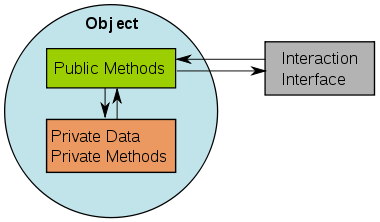# How To Access private member variables of a class without using its public member functions ? Answerred Using C++Today i am going to tell you how to access private member variables of a class without using its public function.

#### Method 1:  Using friend function.

Consider the following example:-

```#include<iostream>
using namespace std;
class A
{
int a;
friend void seta(A &ob,int x);
friend int geta(A &ob);
};
void seta(A &ob,int x)
{
ob.a=x;
}
int geta(A &ob)
{
return ob.a;
}
int main()
{
int x=10;
A obj;
seta(obj,x);
cout<<geta(obj); // 10
return 0;
}```
```OUTPUT:
10```

#### Method 2: Using inheritence.

Consider the following example:-

```#include<iostream>
using namespace std;
class A
{
int a;
protected:
void seta(int x)
{
a=x;
}
int geta()
{
return a;
}
};
class derive:private A
{
public:
void dseta(int x)
{
seta(x);
}
int dgeta()
{
geta();
}
};
int main()
{
int x=10;
derive obj;
obj.dseta(x);
cout<<obj.dgeta(); //10
}```

Here the private variable ‘a’ is a private member of class ‘A’. As condition, class ‘A’ has no public method to access variable ‘a’. I have then created a “derive” class which will inherit class ‘A’ privately. Then i have written public functions on “derive” class. On “main” functions, an object of class “derive” has been created and call its public functions (not base ‘A’ functions). Then those functions will call base class ‘A’ protected functions. Thus the program will have no error and give the correct output.

```OUTPUT:
10```

#### Method 3: Direct Access Using Pointer by type conversion.

Consider the following example:-

```#include<iostream>
using namespace std;
class A
{
int a;
public:
int geta()
{
return a;
}
};
int main()
{
A obj;
int* p = (int*)&obj;
*p = 10;
cout<<obj.geta()<<endl; //10
cout<<*p; //10
}```

This will work on first member variable only and also if data-type of variable and pointer is matched. Pointer will set values on first member variable.

```OUTPUT:
10
10```